## 什么是 R 进制

R 进制数的每一位数值用 $R^k$ 的形式表示，即幂底数为 R，指数为 kk 与该位和小数点间的间距有关。当该位位于小数点左边时，k 则是该位和小数点之间数码的个数；而当该位维语小数点右边时，则 k 是负值，其绝对值为该位和小数点之间数码的个数加 1

## 进制之间的转换

1. 十进制转 R 进制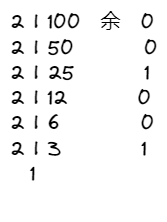1. 二进制转八/十六进制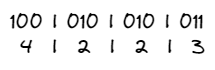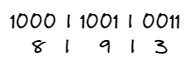## 码制

1 -1 +0 -0

1. 符号位

1. 原码

$0001 + 0010=0011，1 + 2 = 3$

$0000 + 1000= 1000，0 + (-0)=-0$

$0001+1001=1010，1+(-1)=-2$

1. 反码

• 如果一个数是正数，那么其反码和原码一样，如 $1$ 的原码和反码均为 $0001$
• 如果一个数是负数，那么其反码就是其原码除符号位之外，按位取反。如 $-1$ 的原码为 $1001$，其反码为 $1110$

$0001+1001=1111，1+(-1)=-0$

$1110+1100=1010，(-1)+(-3)=-5$

1. 补码

• 如果一个数是正数，那么该数的补码等于其原码，如 $1$ 的原码和补码均为 $0001$
• 如果一个数是负数，那么该数的补码等于反码 $+1$，如 $-1$ 的反码为 1110，那么其补码即为 1111

$1111+1111=1110，(-1)+(-1)=-2$

1. 不同码制之间的转换总结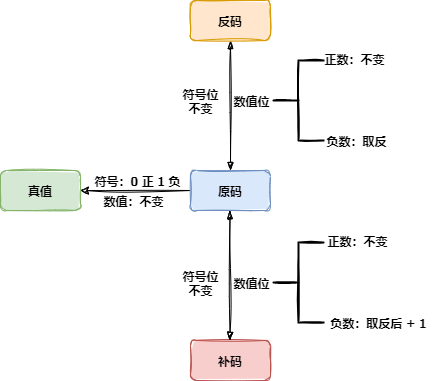1. 移码

1 -1 +0 -0

## 数值表示范围

$-(2^{n-1}-1)$ ~ $+(2^{n-1}-1),-127~+127$

$-(1-2^{-(n-1)})$ ~ $+(1-2^{-(n-1)})$

## 浮点数的运算

### 浮点数的表示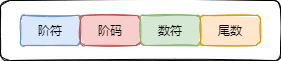$N = M * R^e$

### 浮点数运算

$m = 1.0*10^3$

$n=0.25*10^3$

## 总结